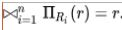# Introduction of 4th and 5th Normal form in DBMS

• Difficulty Level : Hard
• Last Updated : 02 Feb, 2022

Prerequisite – Functional Dependency, Database Normalization, Normal Forms
If two or more independent relation are kept in a single relation or we can say multivalue dependency occurs when the presence of one or more rows in a table implies the presence of one or more other rows in that same table. Put another way, two attributes (or columns) in a table are independent of one another, but both depend on a third attribute. A multivalued dependency always requires at least three attributes because it consists of at least two attributes that are dependent on a third.

For a dependency A -> B, if for a single value of A, multiple value of B exists, then the table may have multi-valued dependency. The table should have at least 3 attributes and B and C should be independent for A ->> B multivalued dependency. For example,

```Person->-> mobile,
Person ->-> food_likes ```

This is read as “person multidetermines mobile” and “person multidetermines food_likes.”

Note that a functional dependency is a special case of multivalued dependency. In a functional dependency X -> Y, every x determines exactly one y, never more than one.

#### Fourth normal form (4NF):

Fourth normal form (4NF) is a level of database normalization where there are no non-trivial multivalued dependencies other than a candidate key. It builds on the first three normal forms (1NF, 2NF and 3NF) and the Boyce-Codd Normal Form (BCNF). It states that, in addition to a database meeting the requirements of BCNF, it must not contain more than one multivalued dependency.

Properties – A relation R is in 4NF if and only if the following conditions are satisfied:

1. It should be in the Boyce-Codd Normal Form (BCNF).
2. the table should not have any Multi-valued Dependency.

A table with a multivalued dependency violates the normalization standard of Fourth Normal Form (4NK) because it creates unnecessary redundancies and can contribute to inconsistent data. To bring this up to 4NF, it is necessary to break this information into two tables.

Example – Consider the database table of a class which has two relations R1 contains student ID(SID) and student name (SNAME) and R2 contains course id(CID) and course name (CNAME).

Table – R1(SID, SNAME)

Table – R2(CID, CNAME)

When there cross product is done it resulted in multivalued dependencies:

Table – R1 X R2

Multivalued dependencies (MVD) are:

` SID->->CID; SID->->CNAME; SNAME->->CNAME`

Joint dependency – Join decomposition is a further generalization of Multivalued dependencies. If the join of R1 and R2 over C is equal to relation R then we can say that a join
dependency (JD) exists, where R1 and R2 are the decomposition R1(A, B, C) and R2(C, D) of a given relations R (A, B, C, D). Alternatively, R1 and R2 are a lossless decomposition of R. A JD ⋈ {R1, R2, …, Rn} is said to hold over a relation R if R1, R2, ….., Rn is a lossless-join decomposition. The *(A, B, C, D), (C, D) will be a JD of R if the join of join’s attribute is equal to
the relation R. Here, *(R1, R2, R3) is used to indicate that relation R1, R2, R3 and so on are a JD of R.

Let R is a relation schema R1, R2, R3……..Rn be the decomposition of R. r( R ) is said to satisfy join dependency if and only ifExample –

Table – R1

`Company->->Product `

Table – R2

`Agent->->Company `

Table – R3

`Agent->->Product `

Table – R1⋈R2⋈R3

`Agent->->Product `

#### Fifth Normal Form / Projected Normal Form (5NF):

A relation R is in 5NF if and only if every join dependency in R is implied by the candidate keys of R. A relation decomposed into two relations must have loss-less join Property, which ensures that no spurious or extra tuples are generated, when relations are reunited through a natural join.

Properties – A relation R is in 5NF if and only if it satisfies following conditions:

1. R should be already in 4NF.

2. It cannot be further non loss decomposed (join dependency)

Example – Consider the above schema, with a case as “if a company makes a product and an agent is an agent for that company, then he always sells that product for the company”. Under these circumstances, the ACP table is shown as:

Table – ACP

The relation ACP is again decompose into 3 relations. Now, the natural Join of all the three relations will be shown as:

Table – R1

Table – R2

Table – R3

Result of Natural Join of R1 and R3 over ‘Company’ and then Natural Join of R13 and R2 over ‘Agent’and ‘Product’ will be table ACP

Hence, in this example, all the redundancies are eliminated, and the decomposition of ACP is a lossless join decomposition. Therefore, the relation is in 5NF as it does not violate the property of lossless join.

My Personal Notes arrow_drop_up